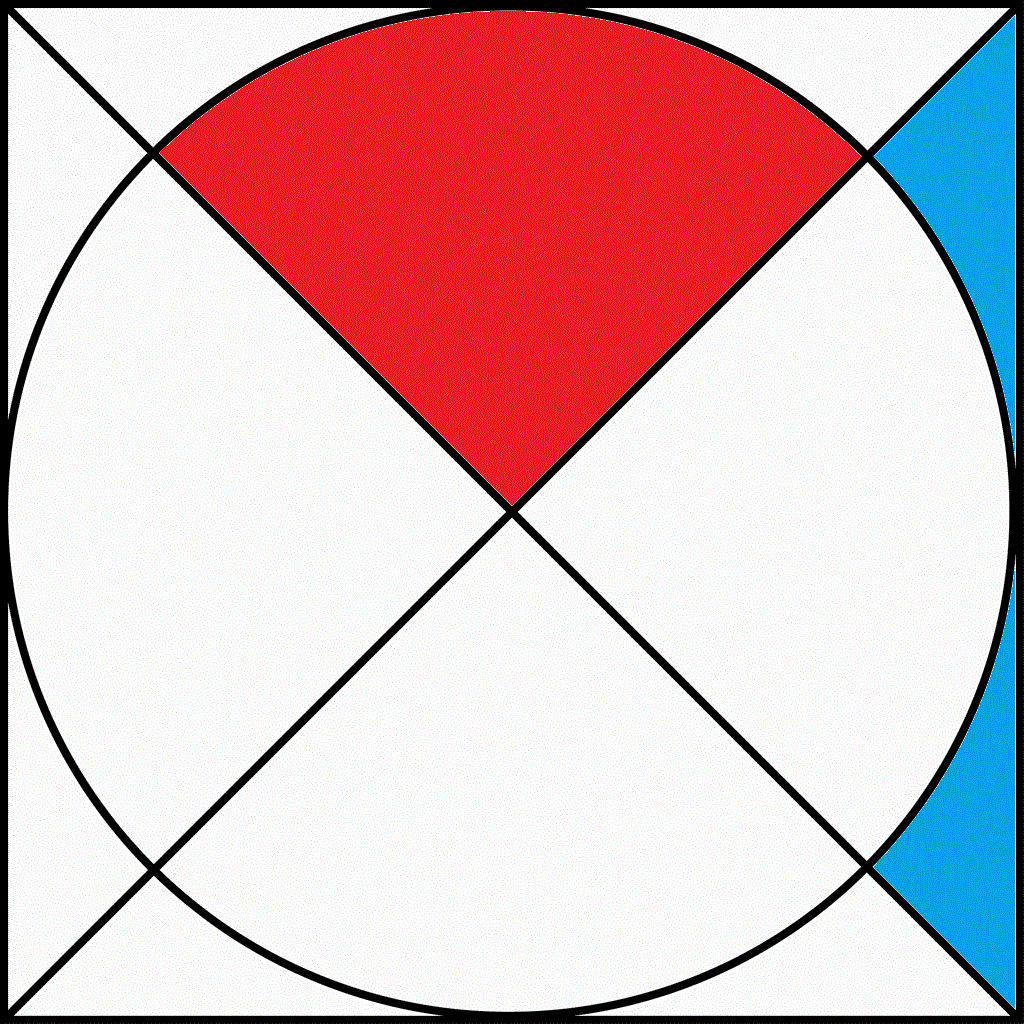# Perfect fit

Geometry Level 2Consider the image above. Let $R$ and $B$ denote the area of the red section and blue section respectively. If the area of the square is $1024 \text{ cm}^2$, find $\frac B R$. Give your answer to 3 decimal places.

Note - The image is up to scale.

×